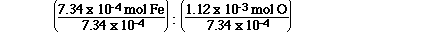### If we use the units grams instead of u's 180 grams is the mass of 1 mol of C6H12O6. And 72 grams of the 180 grams are grams of carbon, 12.1 grams are grams of hydrogen and 96 grams are grams of oxygen. Now we have a very easy way of determining the percentage composition of the elements in C6H12O6.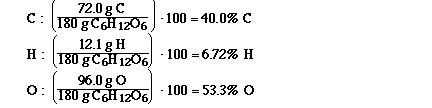### Because by assuming 100 g of the compound you would have 40.0 g of carbon, 6.72 grams of hydrogen and 53.3 grams of oxygen. And now that we have grams of each of the elements it is easy to calculate the number of moles of each element.### Because by assuming 100 g of the compound you would have 26.6 g of potassium, 35.4 grams of chromium and 38.1 grams of oxygen. And now that we have grams of each of the elements it is easy to calculate the number of moles of each element.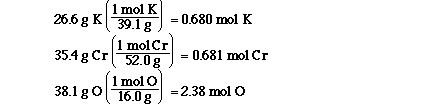### Begin by assuming 100 g of the compound you would have 81.8 g of carbon, and 18.2 grams of hydrogen. Having grams of each of the elements it is easy to calculate the number of moles of each element.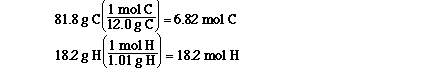### To do this we must divide each of the mol by the smallest number of mol, that will give us our simplest ratio.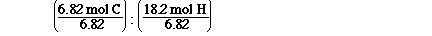### In this case we do not have to assume 100 grams of the compound because we already have the information in grams. The mass of iron is given to us. We are told it is iron oxide so we must determine the mass of oxygen in the compound. we can obtain the mass of oxygen by suybtracting the mass of iron from the mass of iron oxide. When we do this the mass of oxygen is 0.0589 g - 0.410 g = 0.0179 g of O. Having the mass of all of the elements we can determine mol.### To do this we must divide each of the mol by the smallest number of mol, that will give us our simplest ratio.